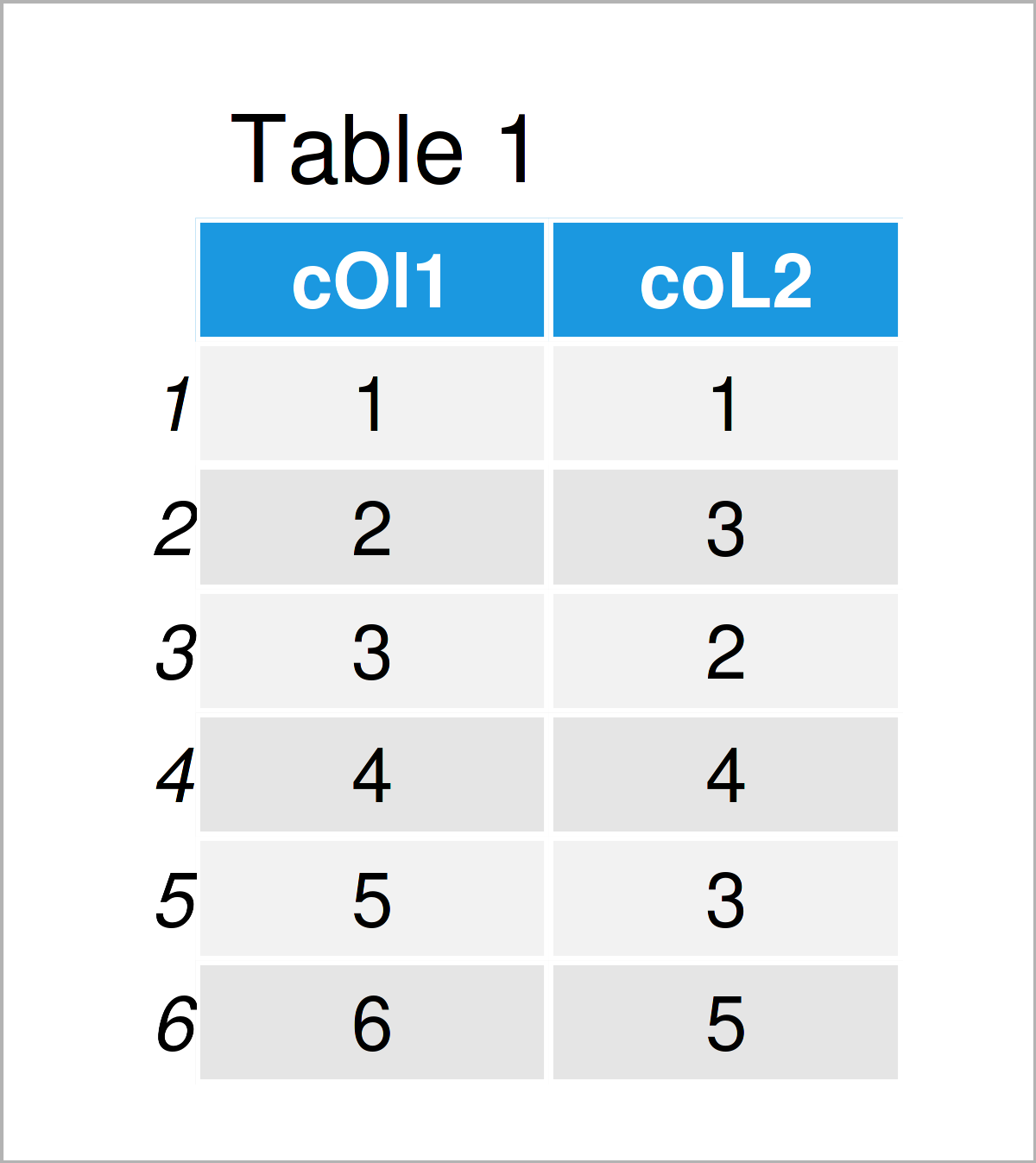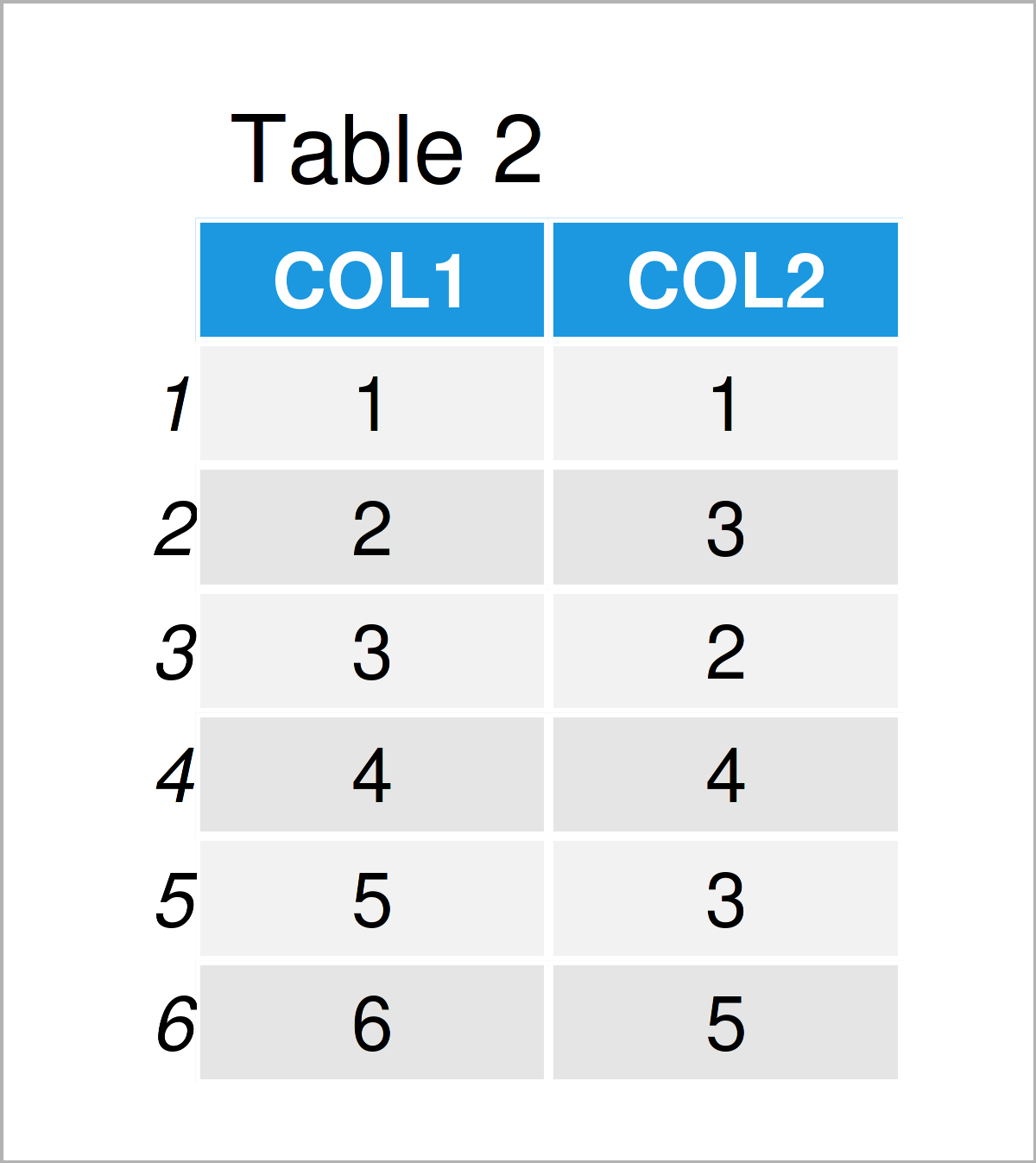# Change Letter Case of Column Names in R (2 Examples)

In this R programming article you’ll learn how to convert variable names to upper and lower case.

The article is structured as follows:

Let’s jump right to the examples!

## Creation of Example Data

As the first step, we’ll need to create some data that we can use in the following example code:

```data <- data.frame(cOl1 = 1:6,         # Create example data frame
coL2 = c(1, 3, 2, 4, 3, 5))
data                                   # Print example data frame```Table 1 visualizes that our example data contains six data points and two columns that are named “cOl1” and “coL2”. The variable cOl1 has the integer class and the variable coL2 is numerical.

Note that both variable names contain upper and lower case letters. Let’s change that!

## Example 1: Change Letters in Column Names to Upper Case

The following R code shows how to capitalize all letters in the column names of a data frame.

For this task, we can apply the names and toupper functions as shown below:

```names(data) <- toupper(names(data))    # Convert colnames to upper case
data                                   # Print updated data frame```In Table 2 it is shown that we have created a new version of our data set where all variable names are written in upper case.

## Example 2: Change Letters in Column Names to Lower Case

The R syntax below illustrates how to switch from upper case to lower case using the tolower function:

```names(data) <- tolower(names(data))    # Convert colnames to lower case
data                                   # Print updated data frame```Table 3 shows the output of the previous R code: A data matrix containing only lower case columns.

## Video, Further Resources & Summary

I have recently released a video on my YouTube channel, which illustrates the content of the present page. Please find the video below:

Also, you may read the related articles on this homepage. I have released numerous other articles already.

This tutorial has explained how to change column names to upper and lower case in R. Tell me about it in the comments section below, if you have additional questions.

Subscribe to the Statistics Globe Newsletter